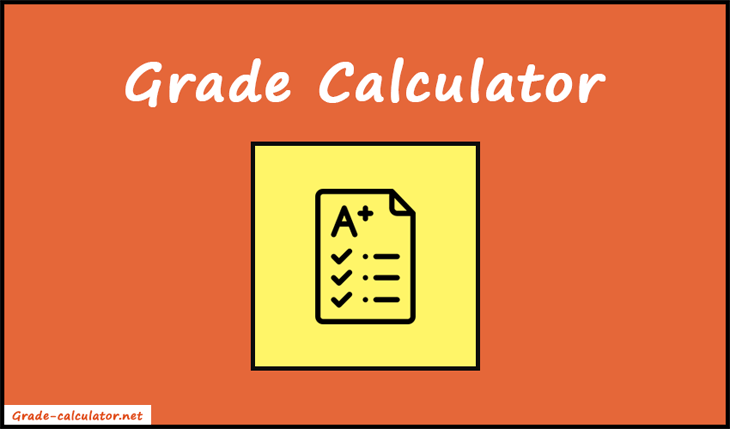Grade Calculator lets you find the weighted percentage and letter grades for all of your assignments or exams.

 Assignment(optional) Grade (letter) Grade (%) Weight -- A+ A A- B+ B B- C+ C C- D+ D D- F -- A+ A A- B+ B B- C+ C C- D+ D D- F -- A+ A A- B+ B B- C+ C C- D+ D D- F -- A+ A A- B+ B B- C+ C C- D+ D D- F -- A+ A A- B+ B B- C+ C C- D+ D D- F Total:
%

Welcome to our Grade Calculator. It's a free and easy-to-use online tool to calculate weighted average grades. In addition, it lets you compute the grades in percentages, letters, or points.

Just select the grade type and input the assignment names, grades, and weights. Lastly, press the "Calculate" button to see the final result.### How to Calculate Weighted Grade?

To calculate a weighted grade, you need to assign different weights or percentages to each assignment. Such as projects, midterms, homework, and quizzes, and then multiply the grade earned in each assignment by its corresponding weight. Finally, add up these weighted grades and divide them by the sum of weights to obtain an overall weighted grade.

 w1×g1 + w2×g2 + w3×g3 + ... w1 + w2 + w3 + ...

Here's an example:

Suppose there are three categories of assignments:

• Projects (weight of 35%)
• Midterms (weight of 40%)
• Homework (weight of 25%)

A student earns the following grades in each category:

• Projects - 75%
• Midterms - 90%
• Homework - 82%

Weighted grade = (project grade × 35%) + (midterm grade × 40%) + (homework grade × 25%) / 35 + 40 + 25

= (75 × 35) + (90 × 40) + (82 × 25) / 100

= (2625 + 3600 + 2050) / 100

= 8275 / 100

= 82.75%

Therefore, the student's overall weighted grade for the course is 82.75%.

### How to use Grade Calculator?

Before getting started, ask your teacher, how much each assignment category is worth. Also, you can check it out from the course syllabus. Because it will be needed for the grade calculation.

1. Firstly, select the grade type. Our tool gives you access to enter the grades in three formats. Percentage, Letters, and Points. So, choose the required one of them.
2. After choosing the grade type, enter the assignment category name. Such as projects, quizzes, homework, midterm, etc.
3. Now enter the grade that you earned for each assignment. Also, you can input the grades in percentages, letters, or points.
4. Enter the weight for each assignment category in percentage. For example, projects - 20%, midterms - 30%, etc.
5. If you want to calculate the grades for more than five assignments, you can use the "Add Assignment" button to add more rows.
6. In addition, you can find how much additional grade is needed to maintain the given average grade. It's an optional feature. You can leave it blank if you don't need it.
7. Lastly, press the "Calculate" button to see the final result on your screen.
8. Finally, you can see the average grade result with calculations and additional grades needed. Also, if you choose the letter grade, you will get the GPA result. Similarly, the tool will display the total grade result, if you choose a point grade.
9. For new calculations, press the "Reset" button. It will refresh the calculator and gives a fresh new start.